# Quiz 6: Economic Growth

Business

Nominal GDP measures the value of all the goods and services at current price manufactured in an economy over a period. Real GDP measures the value of all the goods and services at base year price manufactured in an economy over a period. It measures the performance of an economy. The following information is given about country B: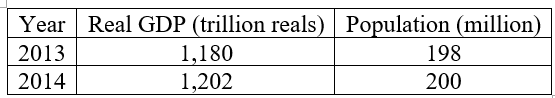a. Compute the growth rate of real GDP as follows:Hence, the growth rate of real GDP is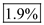. b. To compute the real GDP per person, first, compute the real GDP per person in each year. Compute the real GDP per person in year 2013 and 2014 as follows:Now, compute the growth rate of real GDP per person as follows:Hence, the growth rate of real GDP per person is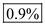. c. The rule of 70 determines the number of years in which a variable gets doubled. The formula of the rule is as follows: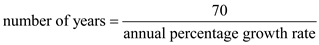Using the above formula, compute the number of years in which the real GDP per person gets doubled as follows: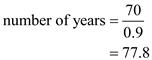Hence, the approximate number of years takes to doubled real GDP per person is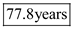.

A rise in the real GDP per capita can be considered as sign of improvement in the standard of living. Note that China's projected value GDP per capita is ¥15040 is 2015 which is expected to reach ¥16010 in 2016. In contrast, India's projected value GDP per capita is 54085 is 2015 which is expected to reach 56840 in 2016. Table 1 encapsulates the growth rate of real GDP per capita for both the countries. From 2015 to 2016, China's real GDP per capita will grow by 6.45 percent while India's of real GDP per capita will show a growth rate of only 5.09 percent. This implies that China will be able to double its standard of living well before India. To know the accurate time period, use the Rule of 70.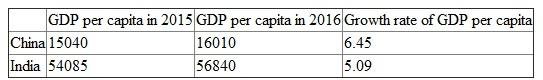Table 1 The rule of 70 entails in finding the approximate number of years after which a sum of money will be doubled, given interest rate. Accordingly, China will take 70/6.45 or 11 years to double its standard of living while India will take 70/5.09 or 13 years to reach the same milestone.

b) America became the world's largest economy in the 1890s because of the Industrial Revolution. At that time, revolution was sweeping Western nations and United states have enjoyed massive resources, economic freedom which leads to them higher productivity and increase the real GDP but China suffered for this revolution and does not accelerate his GDP growth as United Nation. Therefore, increase in economic growth in the U.S, the real GDP become larger than the China's real GDP.